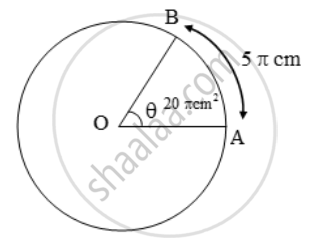Share

# An arc of a circle is of length 5π cm and the sector it bounds has an area of 20 π cm2. Find the radius of the circle. - CBSE Class 10 - Mathematics

ConceptProblems Based on Areas and Perimeter Or Circumference of Circle, Sector and Segment of a Circle

#### Question

An arc of a circle is of length 5π cm and the sector it bounds has an area of 20 π cm2. Find the radius of the circle.

#### Solution

Let the radius of the circle be r cm and the arc AB of length 5π cm subtends angle θ at the centre O of the circle. Then,

Arc AB = 5π cm and

Area of sector OAB = 20π cm2\Rightarrow \frac{\theta }{360}\times 2\pi r=5\pi \text{ and}\frac{\theta }{360}\times \pi r^{2}=20\pi

\Rightarrow \frac{\frac{\theta }{360}\times \pi r^{2}}{\frac{\theta}{360}\times 2\pi r}=\frac{20\pi }{5\pi }

⇒ r/2 = 4 ⇒ r = 8 cm

ALTER: We have, Area = 1/2 /l /r ⇒20π

= 1/2 × 5π × r = 8 cm

Is there an error in this question or solution?

#### Video TutorialsVIEW ALL 

Solution An arc of a circle is of length 5π cm and the sector it bounds has an area of 20 π cm2. Find the radius of the circle. Concept: Problems Based on Areas and Perimeter Or Circumference of Circle, Sector and Segment of a Circle.
S